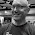## Sunday, January 10, 2021

### Sysbench: in-memory Postgres, Postgres is boring

While writing and rewriting perf reports for MyRocks and InnoDB I have also been running sysbench tests with Postgres. I am happy to claim that Postgres is boring as there are no CPU regressions from version 11 to 13.

I ran tests for Postgres versions 11.10, 12.4 and 13.1 on a small server using sysbench and an in-memory, low-concurrency workload. Workloads with more IO and concurrency are great, but this is the first step. A previous post on how I run these tests. There is one table with 10M rows, tests are run for 1, 2 and 3 clients and each test is run for 180 seconds.

I spent time in 2020 evaluating configuration options for Postgres and settled on the x5 config. Vacuum analyze is run twice -- after the load and again after the write-heavy phase. Along with vacuum analyze, other things are done to write back most of the dirty pages via checkpoint and sleeping (see here).

The summary:

• There are no regressions
• There was a significant (~20%) improvement on one test that is update-only with all updates limited to the same row

Results

The tests are in 5 groups based on the sequence in which they are run: load, read-only run before write-heavy, write-heavy, read-only run after write-heavy and insert/delete.

I have scripts that generate 3 summaries -- absolute throughput, relative throughput and HW efficiency. Absolute throughput is the QPS/TPS that a test gets for 1, 2, and 3 clients. Relative throughput is the QPS/TPS relative to the base case (Postgres 11.10). The HW efficiency report has absolute and relative results (base case is Postgres 11.10) for CPU and IO per operation.

I use ratios to explain performance. In the output files I link to below, Postgres 11.10 is the denominator and the numerator is the value for Postgres 12.4 and 13.1. In my analysis below I focus on Postgres 13.1. A QPS ratio < 1 means the new version is slower. For HW efficiency I use CPU/operation and IO/operation (read & write). For CPU and IO per operation a ratio > 1 means the new version uses more CPU or IO per query.

The results are in github for absolute throughputrelative throughput and HW efficiency. I annotate the relative throughput and HW efficiency results below. Unless called out, I am explaining the results for 13.1 relative to 11.10.

• Inserts/second ratio is 1.08 (~83k/s -> ~90k/s, see here)
• CPU/insert ratio is 0.94 (see here)
• QPS ratios are 1.05, 1.01, 0.97, 0.96 for the first 4 tests (here to here)
• These do point queries
• CPU/query ratios are: 0.96, 0.99, 1.03, 1.06 (here to here)
• QPS ratios are 1.02, 1.01, 0.97 for the next 3 tests (here to here
• These have the range scans from oltp_read_write.lua with ranges of size 10, 100 & 10,000
• CPU/query ratios are 0.99, 0.99, 1.03 (here to here).
• QPS ratios are 0.98, 0.96 for the next 2 tests (here to here)
• These do point queries via in-lists that are covering and then not covering for the PK index
• CPU/query ratios are 1.02, 1.04 (here to here).
• QPS ratios are 0.97, 0.96 for the next 2 tests (here to here)
• These are similar to the previous test, but use the secondary index.
• CPU/query ratios are 1.03, 1.03 (here to here)
• QPS ratios are 1.00, 0.99 for the next 2 tests (here to here)
• These do range queries that are covering and then not covering for the primary index
• CPU/query ratios are 1.00, 1.01 (here to here)
• QPS ratios are 1.01, 1.00 for the next 2 tests (here to here)
• These are similar to the previous test but use the secondary index.
• CPU/query ratios are 0.99, 1.00 (here to here)
Write-heavy
• QPS ratios are 0.99, 0.96, 1.00, 1.20, 1.00 for the next 5 tests (here to here)
• These are update-only. The big gain (1.20) is for a test that does all updates to the same row.
• CPU/statement ratios are 1.01, 1.05, 1.01, 0.86, 1.00 (here to here)
• QPS ratio is 1.00 for the next test, write-only (see here)
• This has the writes from oltp_read_write.lua.
• CPU/transaction ratio is 1.02 (see here)
• QPS ratios are 1.00, 1.01 for the next two tests, read-write (here to here)
• These are the traditional sysbench tests (oltp_read_write.lua) with ranges of size 10 & 100
• CPU/transaction ratios are 0.99, 0.98 (here to here)
Read-only after write-heavy includes tests that were run before write-heavy. The DBMS engines were given a chance to catch up on write back between the write-heavy tests above and the read-only tests below.
• QPS ratio is 1.03, 1.01, 1.00 for the next 3 tests, read-only (here to here)
• These have the range scans from oltp_read_write.lua with ranges of size 10, 100 & 10,000
• CPU/transaction ratios are 0.97, 0.99, 1.00 (here to here)
• QPS ratios are 1.05, 1.01, 0.94, 0.96, 1.00 for the next 5 tests (here to here)
• These do a variety of point queries.
• CPU/query ratios are 0.96, 0.98, 1.06, 1.04, 1.01 (here to here)
• QPS ratios are 0.97, 0.97 for the next 2 tests (here to here)
• These do point queries via in-lists that are covering and then not covering for the PK index
• CPU/query ratios are 1.02, 1.03 (here to here)
• QPS ratios are 0.98, 0.97 for the next 2 tests (here to here)
• These are similar to the previous test, but use the secondary index.
• CPU/query ratios are 0.99, 1.02 (here to here)
• QPS ratios are 1.00, 1.01 for the next 2 tests (here to here)
• These do range queries that are covering and then not covering for the PK index
• CPU/query ratios are 1.00, 0.99 (here to here)
• QPS ratios are 1.00, 1.00 for the next 2 tests (here to here)
• These are similar to the previous test, but use the secondary index.
• CPU/query ratios are 0.99, 1.00 (here to here)
Insert/delete

• QPS ratio is 0.99 for the delete test and 1.02 for the insert test
• CPU/statement ratio is 1.04 for delete and 0.97 for insert

1.1.